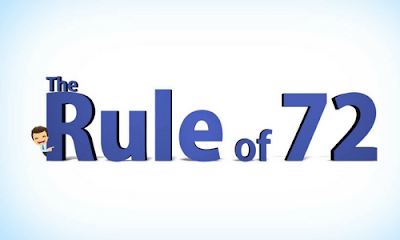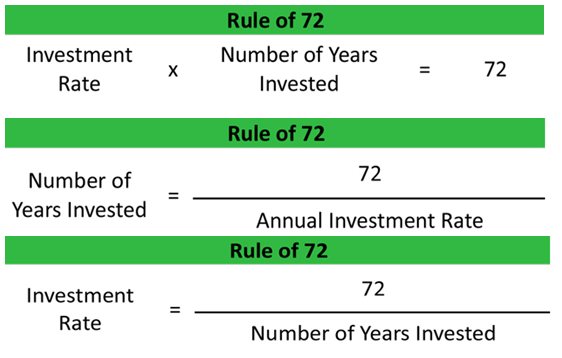# Rule of 72, Rule of 114 and Rule of 144## What is the rule 72?

The rule of 72 is a shortcut technique to estimate the number of years it will take for your money to double with compounding interest.
For example: If you are invested Rs. 100, then how much time it will take to double your money, If the rate of interest is 6%?
So, according to rule of 72:
72 ÷ 6 i.e. 12 years.

Let’s take it in another way: How much time it will take to double a sum of money, if it is invested for 12 years then what should be the rate of interest?
Again, 72 ÷ 12 , i.e 6%

## Following are simple formula:## Following is the table in which the interest is compounded annually:## Following is the table in which different rules are mentioned for Double, triple as well as quadruple:

Rules
Years
4%
5%
6%
7%
8%
9%
10%
11%
12%
Rule of 72
# yrs. to double
18.0
14.4
12.0
10.3
9.0
8.0
7.2
6.5
6
Rule of 114
# yrs. to Triple
28.8
23.0
19.2
16.4
14.4
12.8
11.5
10.5
9.6
Rule of 144
36.0
28.8
24.0
20.6
18.0
16.0
14.4
13.1
12.0

From the above table, we conclude that if the money is double then you need to use the rule of 72. IF the money is triple, then you need to use the rule of 114 and lastly, if the money is quadruple then you need to use the rule of 144.

## Question Asked in RBI GRADE B 2017, in Financial management exam is:

If Rs. 250 is invested today, it will become Rs.1000 in 16 years. What is the compounded rate of interest?
Solution: This is the case of quadruple as the money is increasing four times.
one important thing we need to understand in this is, money is not double here, it is quadruple.
So, simply we use the rule of 144, as mentioned in above table:
144 ÷16 so the answer is 9 years

Examples:-
If Rs. 250 is invested today, it will become Rs.750 in 16 years. What is the compounded rate of interest?
So, simply we use the rule of 114, as mentioned in above table:
114 ÷16 so the answer is 7.12 years

And similarly, If the money is the double i.e rule of 72 is used.
If Rs. 250 is invested today, it will become Rs.500 in 16 years. What is the compounded rate of interest?
72 ÷16 so the answer is 4.5 years.#### What's trending in BankExamsToday

Smart Prep Kit for Banking Exams by Ramandeep Singh - Download here